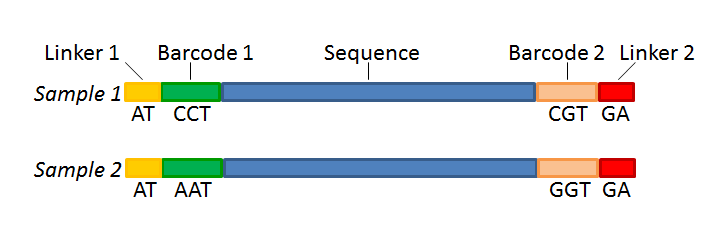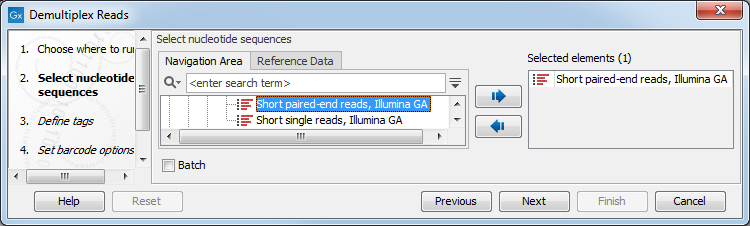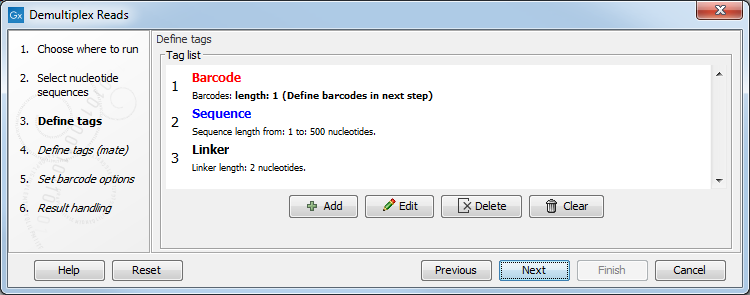If you have paired data the procedure is exactly the same, except for one thing: the dialog shown in figure 25.19 will be displayed twice - one for each part of the pair. Figure 25.22 shows an example that could illustrate paired end reads. In this example we have paired end reads from two different samples mixed together.Figure 25.22: Paired end reads with linkers and barcodes. Two different samples are mixed together in this example.

In a situation where the paired reads are expected to be barcoded in the same way (see example below), you would set the parameters for read1 (wizard step 3) and read2 (wizard step 4) to be the same.

However, if read2 of the pair is not expected to be the same as read1 in the pair, it is necessary to adjust these settings accordingly. For example, it is possible that read2 does not contain any barcode sequence at all. In this case, you would simply set the sequence parameter for the mate and exclude the barcode and linker parameters. If the two reads in the read pair have different barcodes, the situation would look like this: